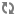Stellar Language Quick Reference# Stellar Language Equality Check

You can use the Stellar language equality check to define both sides of a Stellar syntax.

Below is how the `==` operator is expected to work:

• If either side of the expression is null then check equality using Java's `==` expression.

• Else if both sides of the expression are of Java's type Number then:

• If either side of the expression is a double then use the double value of both sides to test equality.

• Else if either side of the expression is a float then use the float value of both sides to test equality.

• Else if either side of the expression is a long then use long value of both sides to test equality.

• Otherwise use int value of both sides to test equality

• Otherwise use equals method compare the left side with the right side.

The `!=` operator is the negation of the above.